Work Done by a Constant ForceSo, we have discussed the introduction to work and energy in the previous section.

Now let's discuss about work done by a constant force.

Work is defined as the product of the magnitude of the displacement and the component of the force in the direction of the displacement. W=F.d = Fd.cos

Work is a scalar quantity because it is defined as the dot product or scalar product two vectors force and displacement and the scalar product of two vectors gives the scalar quantity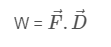﻿Special Cases

1. When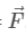and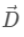are directed in same direction then θ=0. So,
W= FDcos0 =F.D
2. When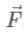and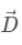are directed in opposite direction, then θ=180. So,
W= FD.cos180 = -F.D
3. Whenandare perpendicular to each other, then θ=90. So,
W= FD.cos90 = 0
So, work done by a force is zero if a body is displaced in a direction perpendicular to the direction of the applied force. For example, if a body is moving in a circle with constant speed, then the centripetal force and the displacement are mutually perpendicular at every point. Therefore, the work done by the centripetal force is zero.

﻿Units and Dimension of Work

﻿We have, W=FD

In SI units, unit of work is Nm or Joules(J). Work done is said to be 1 Joule if a force of 1 Newton displaces a body through 1 meter in the direction of the force.

Also, 1 Joule=1Nm=1N x 1m=105dynes x 100 cm=107 dynes. cm

1 Joule= 107 erg

The dimensional formula of work is [ M  L2  T-2 ]